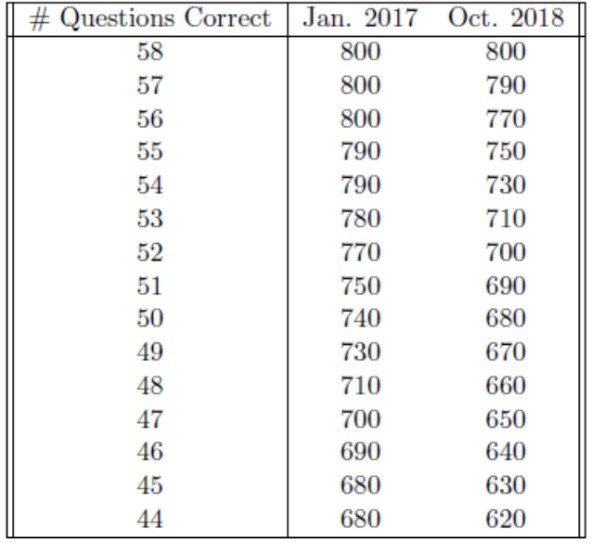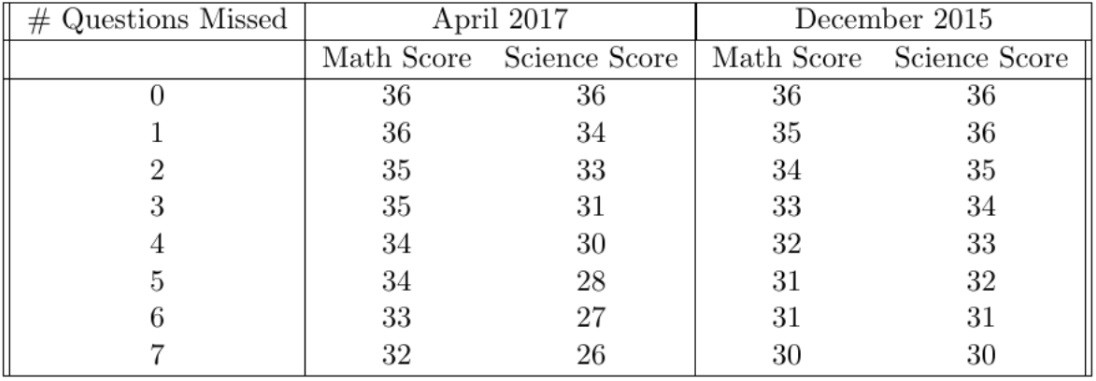Skip to main content

#### Cart

Close Cart

You may have noticed that each administration or test date of the SAT and ACT has a unique scale (or curve) that is distinct from the scales for previous test dates. Students who don’t know this, however, sometimes use the same scale to score different practice tests. This leads to inaccurate scores and, in turn, the potential for misunderstanding one’s strengths and weaknesses.

## Why Do Different Test Dates Have Different Scales?

The answer to why different test dates have different scales is surprisingly straightforward: some tests are easier than others, so it would be unfair to punish or reward students who (simply due to luck) take the test on a date when a particularly easy or hard test is administered. To avoid this, someone who takes an easier test has to get more questions correct than someone who takes a harder test in order to receive the same score.

By doing this, the SAT and the ACT are trying to maximize the reliability of their tests. Theoretically, if the same person takes the test on multiple different test dates, they should receive roughly the same score on the two tests (ignoring changes like studying in between tests, being ill for one of the tests, etc.). In some ways, this is reassuring: regardless of test date, we can be confident that the test is reliably measuring our abilities.

We can see a clear example of this by comparing the scales for the Math sections of the January 2017 and October 2018 SAT exams:To get a 700 in January of 2017, one would have needed to get 47 questions correct. But on the October 2018 test, one would have needed to get 52 questions correct. This is because the October 2018 Math sections were rated as much easier than the January 2017 Math sections.

This may be an extreme example, but it illustrates some clear conclusions. First, when we score a practice SAT or practice ACT, we need to make sure that we are using the scale that is specific to that test. Second, it is probably not useful to think about how many questions we can miss or how many questions we need to get correct on any given section, since this will change from one test to the next.

## What about the Different Sections?

It is totally possible for a particular test to have sections that differ in terms of their difficulties. A test may have a particularly difficult Math section, a Reading section of easier-than-average difficulty, and a Science section of average difficulty. Many other combinations are, of course, possible as well. For example, let’s look at the ACT Math and Science scales from the April 2017 test and the December 2015 test:We can see that the April 2017 test had the harder Math section of the two tests—students could miss more questions in the April 2017 test than in the December 2015 test in order to get the same Math score. For example, to get a 34 on the Math section, the student could miss 5 questions in April 2017 but only 2 questions in December 2015.

The reverse is true for the Science sections.  The April 2017 Science section was rated as being far easier than average, so students could not miss very many questions in order to achieve a high score. If a student missed 5 questions in April 2017, he or she would have received a 28, while the same number of missed questions would have earned 32 in December 2015.

## How Do ACT and SAT Determine the Scales?

For each question that they ask, ACT and SAT use an advanced statistical technique called Item Response Theory to assess the difficulty of the question and how well the question measures the student’s ability or knowledge. Their goal is that an individual would receive the exact same score regardless of which test the individual takes.

Let’s imagine an individual at the 70th percentile of Math ability (i.e., that person has more Math ability than 70% of the other people who take the test). One day, he takes a hard ACT Math section and misses 29 questions, yielding a score of 23. The next day, he takes a much easier Math section and only misses 22 questions. At first, he may be excited about the appearance of very sudden improvement…until he checks the scales and realizes that this is still a score of 23.

This result is exactly what ACT wants: test takers with the same ability level getting the same score, regardless of which version of the test they receive. This is fair for test-takers, and it makes the test a reliable measure of ability. This goal is achieved through “test equating.” Using Item Response Theory, the makers of the ACT and the SAT can estimate what a person of any given ability level would be likely to score on that test section. They can then set the scales for each test so that individuals of that particular ability level always receive the same score. Thus, it isn’t just about how many questions you miss, it’s more about the combination of how many questions you miss and how hard the section is.

## Remember:

We never know ahead of time whether we’re going to get a hard section or an easy section, so our goal should always be simply to get as many questions correct as we can, rather than aiming for a specific number of questions correct or incorrect. Don’t overthink the curve on a test that hasn’t even been graded yet, just focus on applying great process to each question and do the best you can!